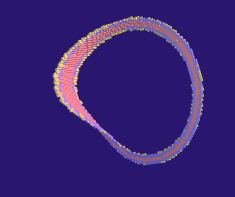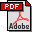# Periodic Orbits

The aim of this research is to develop an automated procedure for proving the existence of periodic trajectories in dynamical systems. The key idea is to construct an isolating neighborhood which would give rise to an index pair for the Conley index. Algorithmic homology computation of this Conley index together with some other rigorous numerical verifications would lead to a mathematically accepted proof of the existence of such a periodic trajectory. Some results of this research have already been published, some other work is still in progress.

## Stable Periodic Trajectories

In the case of an attracting periodic trajectory, an isolating neighborhood itself can be used as an index pair with the empty exit set. Theory and applications in such a situation have been described in the following papers:

• P. Pilarczyk, Computer assisted method for proving existence of periodic orbits, TMNA 1999 Vol. 13 No. 2, 365-377.• P. Pilarczyk, Computer assisted proof of the existence of a periodic orbit in the Rössler equations, Proceedings of the International Conference on Differential Equations (Berlin, 1999), 228-230, World Sci. Publishing, Singapore, 2000.• M. Mrozek, P. Pilarczyk, The Conley index and rigorous numerics for attracting periodic orbits, Proceedings of the Conference on Variational and Topological Methods in the Study of Nonlinear Phenomena (Pisa, 2000), 65-74, Progr. Nonlinear Differential Equations Appl., 49, Birkhäuser Boston, Boston, MA, 2002.## Unstable Periodic Trajectories

The case of unstable periodic orbits is much more demanding, not only because an index pair with a non-empty exit set must be constructed, but also due to numerical issues: Since unstable directions are present in the dynamical system, numerical bounds for rigorously computed trajectories tend to grow exponentially, making it very difficult or even impossible to integrate the underlying differential equation for prolonged periods of time. Some specific periodic orbits have been addressed with success, though, and these cases are described in the following papers:

• P. Pilarczyk, Topological-numerical approach to the existence of periodic trajectories in ODEs, Discrete and Continuous Dynamical Systems 2003, A Supplement Volume: Dynamical Systems and Differential Equations, 701-708.• P. Pilarczyk, The Conley index and rigorous numerics for hyperbolic periodic trajectories in ODEs, in preparation.

## Homology Computation

Since the method used in this research requires one to compute the homology of an index map, suitable algorithms for homology computation must have been developed. The algorithms available in the book

• T. Kaczynski, K. Mischaikow, M. Mrozek, Computational homology, Applied Mathematical Sciences 157, Springer-Verlag, New York, 2004.
turned out to be insufficient, mainly because they do not address the issue of relative homology, obviously necessary for the computation of the homological Conley index map. Therefore, in addition to the reduction algorithms introduced in one of the above-mentioned papers, additional research had to be carried out, which resulted in the following publication:

## Excision Preserving Maps

The definition of a cubical index pair with respect to a discrete dynamical system used in this research has been chosen in a way that seems to be optimal from the point of view of rigorous numerical computations which appear to be the most difficult part of the method. However, due to delicate isolating properties of such an index pair, in some cases difficulties emerge with the index map computation, because the cubical enclosure of the underlying continuous map may loose the excision property of that map. In order to overcome this difficluty, an alternative approach to the index map is proposed in the following paper:

## Parallel Computing

In order to speed up the process of constructing an index pair, a concurrent version of the algorithm introduced in one of the previous papers is beeing developed. It will be described in the following paper:

• P. Pilarczyk, A concurrent algorithm for the construction of index pairs, in preparation.